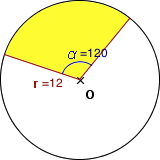# Finding the Area of a Sector: Formula & Practice Problems

Instructions:

Choose an answer and hit 'next'. You will receive your score and answers at the end.

question 1 of 3

### What is the area of the following sector? (approximate pi to 3.14)Create Your Account To Take This Quiz

As a member, you'll also get unlimited access to over 75,000 lessons in math, English, science, history, and more. Plus, get practice tests, quizzes, and personalized coaching to help you succeed.

Try it risk-free for 30 days. Cancel anytime.

### 2. If a clock's face measures 14 inches across, how much area is between the minute and hour hands at 5 o' clock? (approximate pi to 3.14)

Create your account to access this entire worksheet
A Premium account gives you access to all lesson, practice exams, quizzes & worksheets
Quizzes, practice exams & worksheets
Certificate of Completion
Create an account to get started

In this quiz, you will practice finding the area of a sector and finding the angle of a sector through various word problems.

## Quiz & Worksheet Goals

This quiz will test your abilities in:

• Finding the area of a sector given a diagram
• Using information about a sector's area to find the angle

## Skills Practiced

• Knowledge application- use your knowledge to answer questions about finding the angle of a sector
• Making connections- use your understanding of circle diagrams to find the area of a sector in a circle
• Problem solving - use acquired knowledge to solve multiple sector area word problems

If you need more information on this topic, refer to the lesson titled Finding the Area of a Sector: Formula & Practice Problems. This lesson was designed to cover these objectives:

• Define what a sector is
• Understand where a sector originates within a circle
• Describe how to find the area of a sector
• Identify the formula when the angle is provided in degrees
• Know the formula using radians for angles
Final ExamNY Regents Exam - Geometry: Help and Review
Status: Not Started
Chapter ExamNY Regents - Circular Arcs and Circles: Help and Review
Status: Not Started

Support